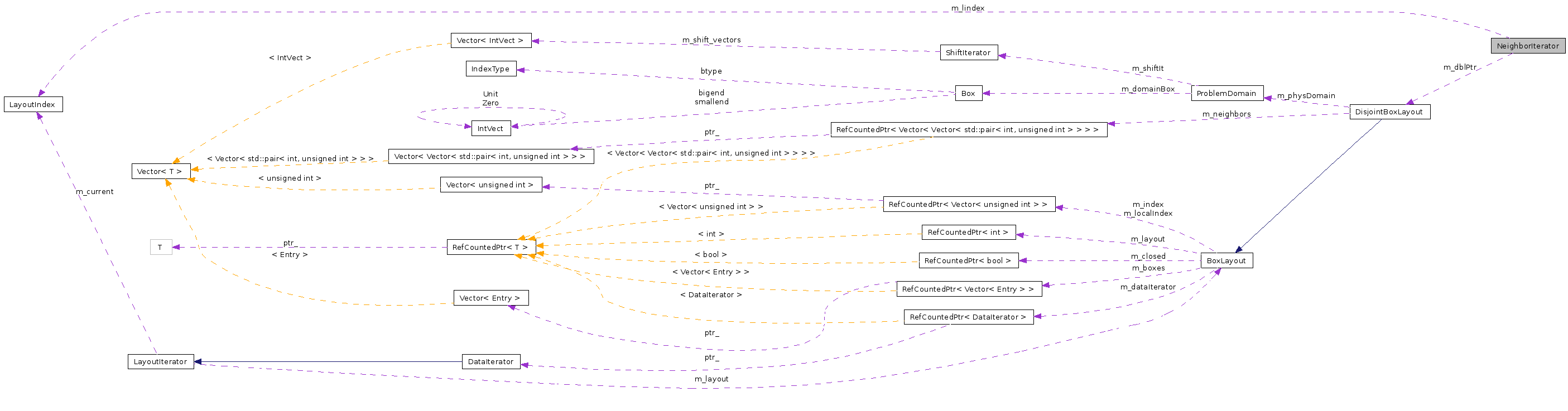# NeighborIterator Class Reference

`#include <NeighborIterator.H>`

Collaboration diagram for NeighborIterator:[legend]

## Detailed Description

An Iterator based on a DisjointBoxLayout object for neighboring boxes.

## Public Member Functions

NeighborIterator ()
NeighborIterator (const DisjointBoxLayout &dbl)
const LayoutIndexoperator() () const
return the index that this iterator is at
Box box () const
void operator++ ()
move the iterator to the next index in the neighbor list
bool ok () const
return true if this iterator is still in the neighbor list
void begin (const DataIndex &a_dataIndex)
initialize this iterator to the first index in the neighbor list
Box unshift (const Box &a_box) const
OK, this one requires a little explanation.

## Private Attributes

const DisjointBoxLayoutm_dblPtr
std::vector< std::pair< int,
unsigned int >
>::const_iterator
m_current
std::vector< std::pair< int,
unsigned int >
>::const_iterator
m_end
LayoutIndex m_lindex

## Constructor & Destructor Documentation

 NeighborIterator::NeighborIterator ( ) ` [inline]`

 NeighborIterator::NeighborIterator ( const DisjointBoxLayout & dbl )

## Member Function Documentation

 const LayoutIndex & NeighborIterator::operator() ( ) const` [inline]`

return the index that this iterator is at

Aborts if the iterator is not ok()

References m_dblPtr, m_lindex, and ok().

 Box NeighborIterator::box ( ) const

 void NeighborIterator::operator++ ( ) ` [inline]`

move the iterator to the next index in the neighbor list

References m_current, m_end, LayoutIndex::m_index, and m_lindex.

 bool NeighborIterator::ok ( ) const` [inline]`

return true if this iterator is still in the neighbor list

References m_current, m_dblPtr, and m_end.

Referenced by operator()().

 void NeighborIterator::begin ( const DataIndex & a_dataIndex )

initialize this iterator to the first index in the neighbor list

 Box NeighborIterator::unshift ( const Box & a_box ) const

OK, this one requires a little explanation.

if the *current* box is a periodic image box, then this operation returns the unmapping of a_box.

## Member Data Documentation

 const DisjointBoxLayout* NeighborIterator::m_dblPtr` [private]`

Referenced by ok(), and operator()().

 std::vector >::const_iterator NeighborIterator::m_current` [private]`

Referenced by ok(), and operator++().

 std::vector >::const_iterator NeighborIterator::m_end` [private]`

Referenced by ok(), and operator++().

 LayoutIndex NeighborIterator::m_lindex` [private]`

Referenced by operator()(), and operator++().

The documentation for this class was generated from the following file:

Generated on Tue Apr 14 14:23:45 2009 for Chombo + EB by1.5.5